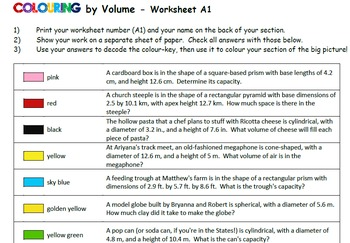# Colouring by Volume, Easter Bunny (Prisms, Pyramids, Cylinders, Cones, Spheres)7th - 12th
Subjects
Standards
Resource Type
Formats Included
• Zip
Pages
50 pages

### Description

Highly engaging! Each student's worksheet is different, ensuring individual accountability. Each worksheet represents a small section of the big picture, providing collaborative motivation! Engage your class in a 25-sheet Easter-themed mosaic involving real-life volume application problems (word problems).

The Easter Bunny mosaic is also available in a Trigonometry Bundle (Right, Acute, Pythagorean Theorem) . These versions can mix 'n match with this one!

Every worksheet contains the following 15 types of questions:

1) volume of rectangular prism
2) volume of rectangular pyramid
3) volume of cylinder
4) volume of cone
5) volume of rectangular prism
6) volume of sphere
7) volume of cylinder
8) volume of rectangular prism
9) volume of sphere given circumference
10) volume of rectangular prism
11) volume of cone
12) volume of cylinder given circumference
13) volume of rectangular pyramid
14) volume of rectangular pyramid
15) volume of cone given circumference

Worksheets integrate metric and imperial units, and use decimal and fractional length values (randomly 20% of the time).

Units are chosen randomly for each problem, providing an opportunity for students to decide if the measurement is realistic or not, building a strong conceptual understanding of cubic units of measurement.

CHECK OUT THE PRODUCT PREVIEW to see sample worksheets, showing the range of problem types. You will know exactly what these worksheets involve!

It's simple!
2. Colour the squares.
4. Combine with the class!

Students complete the problems to decode the colour-by-number key, then colour their section of the mosaic.

INCLUDED:
.pdf and .docx of everything
-A Teaching Tips page
-An answer-range key for quick student assessment at a glance. (i.e. "Blue always has an answer between 120 and 160")
-A coloured coordinate-labelled image of the mosaic to help you assemble the completed picture.
-The "Problem Order" list (shown above), giving the order of problem types on the worksheets.

THE MATH INVOLVED:
-Interpret V word problems arising from real life contexts
-Use volume formulas for cylinders, prisms, pyramids, cones, and spheres to solve problems
-Work with fractional and decimal edge lengths in the context of solving real-world problems
-Use both metric and imperial units of measurement for length and volume, and consider the appropriateness of the (randomly chosen) unit for each problem.
-Use the Pythagorean theorem to solve for necessary dimensions (slant height, perpendicular height, apex height)
-Determine desired dimensions given information about the shape (i.e. get radius from circumference)

LOOK AT THE PREVIEW for the best glimpse at the types of problems involved.

Older kids like colouring too! Their curiosity and desire to see the big picture come together will keep them working and helping each other!

If you like this product, be sure the check out the whole Collaborative Math Mosaic directory, sorted by topic!

All my "Colouring by..." math mosaics use the standard colours found in a Crayola 24 pack of coloured pencils. For best results, use the exact colour name match, and stick to one type of colouring medium. Maybe a class set of pencil crayons would be a fun departmental purchase? :)

I'd love to hear from you! Leave some feedback through TpT about how this went in your classroom, or email me at calfordmath@live.ca.
Total Pages
50 pages
Included
Teaching Duration
3 days
Report this Resource to TpT
Reported resources will be reviewed by our team. Report this resource to let us know if this resource violates TpT’s content guidelines.

### Standards

to see state-specific standards (only available in the US).
Know the formulas for the area and circumference of a circle and use them to solve problems; give an informal derivation of the relationship between the circumference and area of a circle.
Solve real-world and mathematical problems involving area, volume and surface area of two- and three-dimensional objects composed of triangles, quadrilaterals, polygons, cubes, and right prisms.
Apply the Pythagorean Theorem to determine unknown side lengths in right triangles in real-world and mathematical problems in two and three dimensions.
Know the formulas for the volumes of cones, cylinders, and spheres and use them to solve real-world and mathematical problems.
Use volume formulas for cylinders, pyramids, cones, and spheres to solve problems.